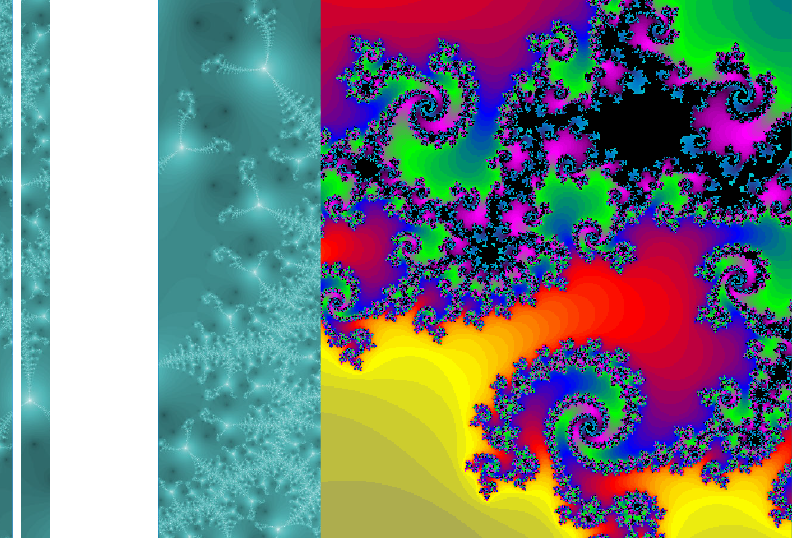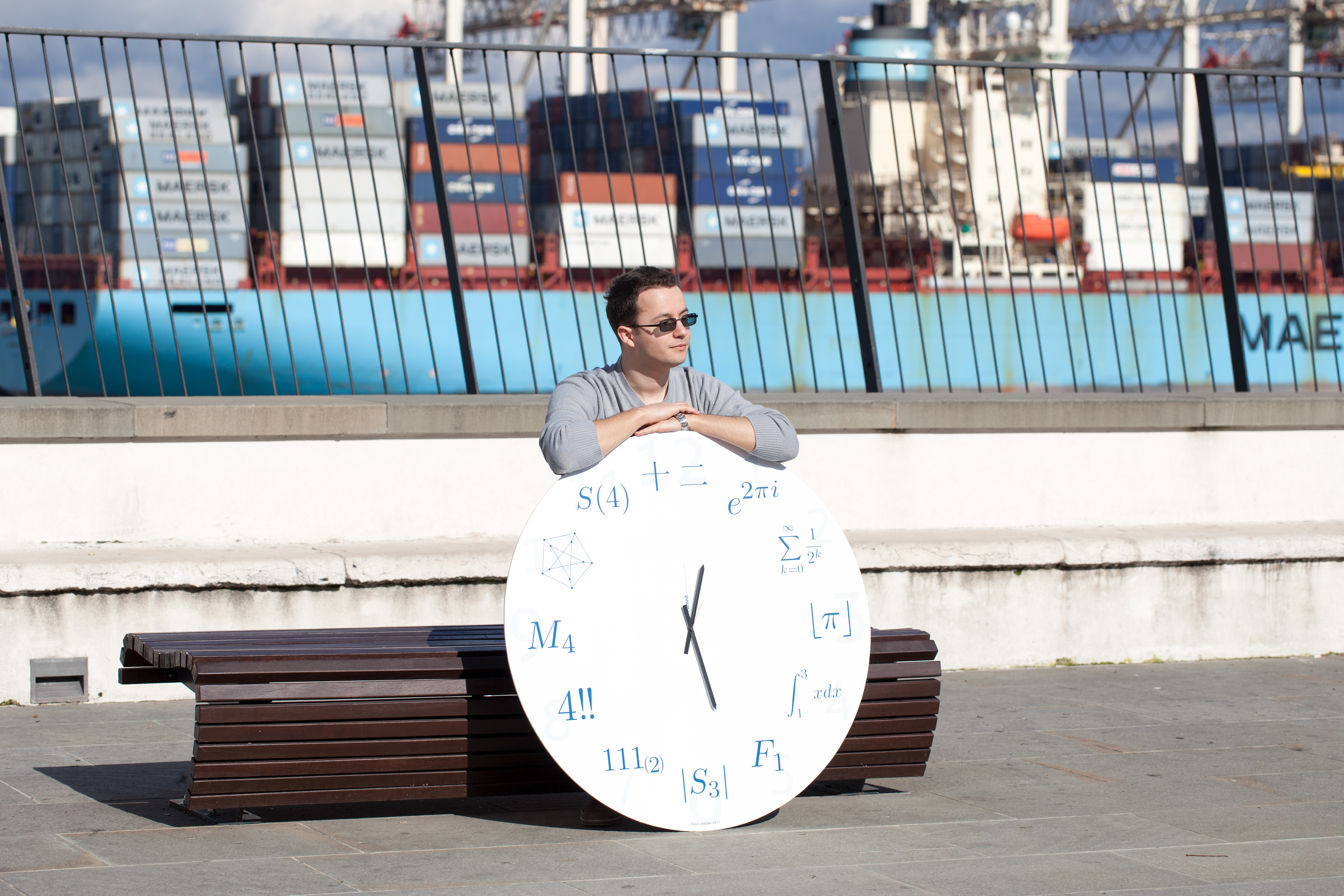Univerza na Primorskem Fakulteta za matematiko, naravoslovje in informacijske tehnologijeSI | EN

# 2013 PhD Summer School in Discrete Mathematics - Minicourse II

natisni

MINICOURSE 2: Leonard pairs and the q-Racah polynomials

Speaker: Prof. Tatsuro Ito, Kanazawa University, Japan

Abstract:

A dual system of orthogonal polynomials arises from the Bose- Mesner algebra of an association scheme that is metric and cometric (or P- and Q-polynomial in Delsarte-Bannai’s term). D. Leonard classified such orthogonal polynomials and they turned out to be in one to one correspondence with the q-Racah polynomials or certain limits of these polynomials.

P. Terwilliger interpreted the above dual system of orthogonal polynomials as two linear transformations each acting in an irreducible tridiagonal fashion on an eigenbasis of the other one. He called such linear transformations a Leonard pair. He classified them and established a representation theory for them. This immediately leads to a classification of dual systems of orthogonal polynomials as well as a characterization of the q-Racah polynomials including their limiting cases.

The theme of my lectures will be Terwilliger’s theory of Leonard pairs. After a brief introduction to the relation between a Leonard pair and a dual system of orthogonal polynomials, I will introduce a raising map R and a lowering map L via the split decomposition (weight- space decomposition) attached to a Leonard pair. The eigenvalues of the Leonard pair will be written explicitly by the Askey-Wilson parameters. The key here is the tridiagonal relations (TD-relations). It turns out that the TD-relations nearly characterize Leonard pairs.

I will then introduce pre-Leonard pairs, relaxing the conditions for Leonard pairs, and ask when a pre-Leonard pair is a Leonard pair. The keys here are Terwilliger’s lemma and the Askey-Wilson relations. We show that a pre-Leonard pair is a Leonard pair if and only if the data of the pre-Leonard pair, i.e., the eigenvalues together with the local traces of LR, allow Askey-Wilson parametrizations. This estasblishes a bijection between the set of data and the isomorphism classes of Leonard pairs. It also gives a way to construct a Leonard pair for each admissible data.

Finally I will explicitly write down dual systems of orthogonal polynomials as q-Racah polynomials and explain the limiting cases.